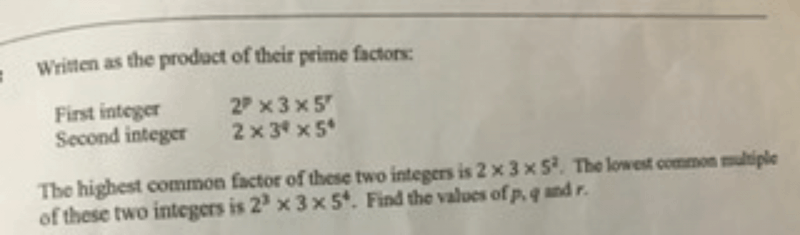# Question0 Replies 0 Likes

1st integer: 2p × 3 × 5r
2nd integer: 2 × 3q × 54

HCF: 2 × 3 × 52
LCM: 23 × 3 × 54

To find the HCF between two numbers using prime factorisation method, we take the prime factors which have the lower power, and to find LCM, we take the one with the higher power.

Therefore, just by looking at the LCM we can tell that p = 3 and q = 1. Looking at the HCF, we can tell that r is 2.

To get the HCF of two (or more) numbers after having expressed them as a product of their prime factors, we simply need to compare them. As an example, let’s try to get the HCF of 150 and 15,000.

150 = 2 × 3 × 52
15,000 = 23 × 3 × 54

From the above, we can see that 150 and 15,000 both have some “2”s and “3”s and “5”s which make up their prime factors. To find the HCF, we simply need to compare them and multiply only the smaller ones / the ones with a lower exponent. This means that we take the 21 from 150 instead of the 23 from 15,000. Since the next factor 3 has the same exponent, it doesn’t matter which one we take. As for the factor 5, we take the 52 from 150 instead of the  54 from 15,000.

Multiplying them, we have 2 × 3 × 52 = 150. This is the HCF.

But what about LCM? We still express the numbers as a product of their prime factors and compare them, but this time, we multiply the larger of the factors / the ones with the larger exponent. This means that we take 23 from 15,000 instead of the 21 from 150. The next factor 3 has the same exponent, so it doesn’t matter which one we take. As for the factor 5, we take the 54 from 15,000 instead of the 52 from 150.

Multiplying them, we have 23 × 3 × 54 = 15,000. This is the LCM.

This method is called the prime factorisation method, and that was how I could tell the values of p, q, and r just by looking at the HCF and LCM given. Hope this is clear.

0 Replies 0 Likes